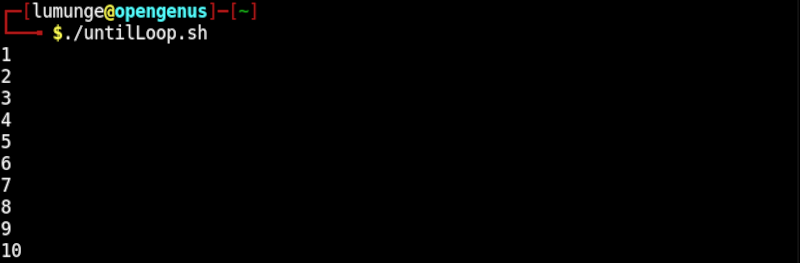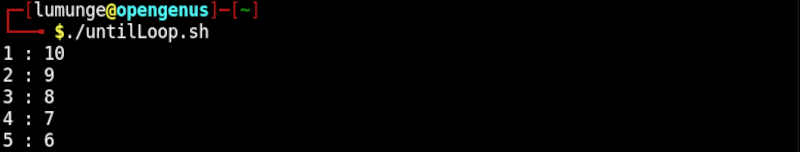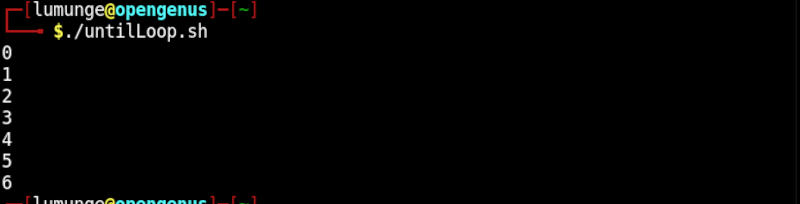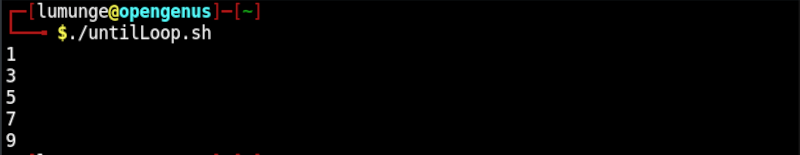×

Search anything:

# Bash until loop

#### Linux Software EngineeringGet this book -> Problems on Array: For Interviews and Competitive Programming

Loops are an important aspect of any programming language. In this article, we look at the until loop that is used for looping in shell scripts.

1. Introduction.
2. Syntax.
3. Examples.
4. Summary.
5. References.

## Introduction.

Looping is an important part of any programming. In shell scripting, we have three types of loops for loops, while loops, and until loops. We have covered for and while loops in the prerequisite article.

Unlike the while loop that executes as long as some condition is true, the until loop assumes the condition is false and thus executes until the condition changes to true.
An example use case is searching for data, we could loop until the search results reach a certain threshold, we could also create a script that executes until a user enters exit.

## Syntax.

The until the loop is written as follows;

``````until [condition]; do
statements
done
``````

## Examples.

1. In this example we print integers from 1 to 10. We have a variable x which we have initialized as 1. The condition is as follows: Until x is equal to 10, we will print out its value. To avoid an infinite loop, we will increment the value of x by 1 after each iteration.

The code is as follows;

``````#! /bin/bash

x=1

# this is an infinite loop
until [ \$x == 10 ]; do
echo "\$x";
x=\$((x + 1))
done
echo "\$x"
``````

The output:We can also pass multiple conditions however, we have to use the following syntax;

``````until [[ expression ]]; do
statement(s)
done
``````
1. In this example we have two variables, i whose value is 1 and j whose value is 10. The loop states that until the value of i is greater than 4 and the value of j is less than 6, print their values. To prevent an infinite loop we increment i by 1 and decrement j by 1.
``````#! /bin/bash

i=1
j=10

# this is an infinite loop
until [[ \$i > 4 && \$j < 6 ]]; do
echo "\$i : \$j";
i=\$((i + 1))
j=\$((j - 1))
done
``````

We have the following output;1. Until loop with break statement.
The break statement is used to terminate the loop and pass control to the next statement after it.
We use break statements with until loops as follows;
``````#! /bin/bash

i=0

until [ \$i -eq 10 ]; do
if [ \$i -eq 7 ]
then
break
fi
echo "\$i";
i=\$((i + 1))
done
``````

First, we initialize variable i to 0. The loop states that until the value of i is equal to 10, echo the value of i. Within the loop we have a statement that checks if the value of i is equal to 7. If it is, the loop terminates and passes control to the next statement.

In this case, we have no following statements therefore we have the following output:1. Until loop with continue statement.
The continue statement exits the current iteration of the loop so the next one can begin.
``````#! /bin/bash

i=0

until [ \$i -eq 10 ]; do
i=\$((i + 1))
[ \$((i%2)) -eq 0 ] && continue
echo "\$i";
done
``````

In the above code, we initialize i to 0. The loop states that until the value of i is equal to 10 we print the value of i. We also increment the value of i in each iteration. Within the loop we check if the remainder of the division between i and 2 is equal to zero, that is, we check if the value of i at each iteration is even. If it is, we exit the current iteration, meaning that we don't execute the following statement but instead control jumps to the beginning of the loop for the next iteration.
We have the following output.## Summary.

It is inevitable to use loops in any programming that involves processing multiple values this includes bash scripting, looping can be used to iterate over files in a file system or iterate over logs or command output.

In this article, we have seen how to use the until loop in bash scripts.

#### Erick Lumunge

Erick is a passionate programmer with a computer science background who loves to learn about and use code to impact lives positively.